# Grid Unique Paths : DP on Grids (DP8)

Given two values M and N, which represent a matrix[M][N]. We need to find the total unique paths from the top-left cell (matrix) to the rightmost cell (matrix[M-1][N-1]).

At any cell we are allowed to move in only two directions:- bottom and right.

```Example: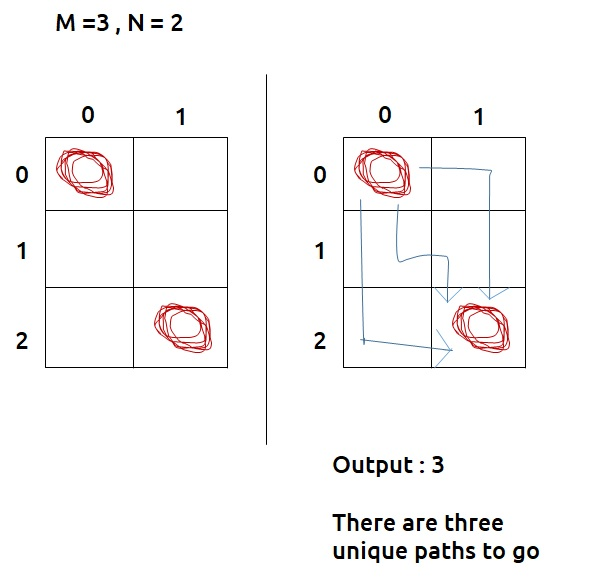```

Disclaimer: Don’t jump directly to the solution, try it out yourself first.

Pre-req: Patterns in Recursion

### Solution :

As we have to count all possible ways to go from matrix[0,0] to matrix[m-1,n-1], we can try recursion to generate all possible paths.

Steps to form the recursive solution:

We will first form the recursive solution by the three points mentioned in Dynamic Programming Introduction

Step 1: Express the problem in terms of indexes.

We are given two variables M and N, representing the dimensions of a 2D matrix. For every different problem, this M and N will change.

We can define the function with two parameters i and j, where i and j represent the row and column of the matrix.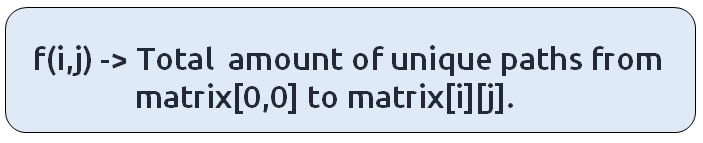f(i,j) will give us a sub-answer for the region (marked in blue) below: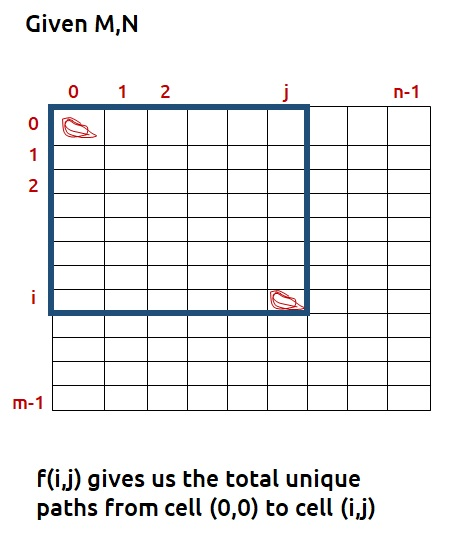We will be doing a top-down recursion, i.e we will move from the cell[M-1][N-1] and try to find our way to the cell. Therefore at every index, we will try to move up and towards the left.

Base Case:

As discussed in  Patterns in Recursion, there will be two base cases:

• When i=0 and j=0, that is we have reached the destination so we can count the current path that is going on, hence we return 1.
• When i<0 and j<0, it means that we have crossed the boundary of the matrix and we couldn’t find a right path, hence we return 0.

The pseudocode till this step will be: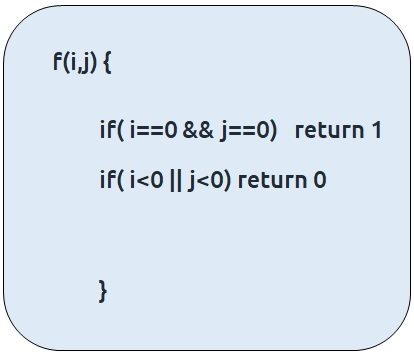Step 2: Try out all possible choices at a given index.

As we are writing a top-down recursion, at every index we have two choices, one to go up(↑) and the other to go left(←). To go upwards, we will reduce i by 1, and move towards left we will reduce j by 1.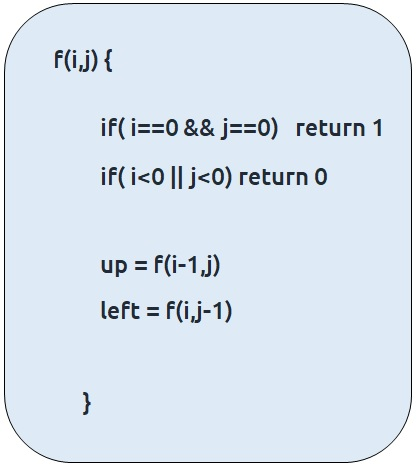Step 3:  Take the maximum of all choices

As we have to count all the possible unique paths, we will return the sum of the choices(up and left)

The final pseudocode after steps 1, 2, and 3: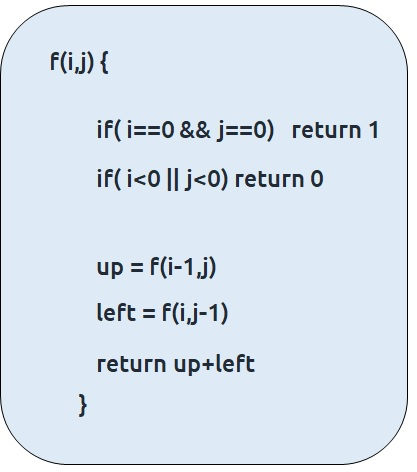Steps to memoize a recursive solution:

If we draw the recursion tree, we will see that there are overlapping subproblems. In order to convert a recursive solution the following steps will be taken:

1. Create a dp array of size [m][n]
2. Whenever we want to find the answer of a particular row and column (say f(i,j)), we first check whether the answer is already calculated using the dp array(i.e dp[i][j]!= -1 ). If yes, simply return the value from the dp array.
3. If not, then we are finding the answer for the given values for the first time, we will use the recursive relation as usual but before returning from the function, we will set dp[i][j] to the solution we get.

Code:

## C++ Code

``````#include <bits/stdc++.h>

using namespace std;

int countWaysUtil(int i, int j, vector<vector<int> > &dp) {
if(i==0 && j == 0)
return 1;
if(i<0 || j<0)
return 0;
if(dp[i][j]!=-1) return dp[i][j];

int up = countWaysUtil(i-1,j,dp);
int left = countWaysUtil(i,j-1,dp);

return dp[i][j] = up+left;

}

int countWays(int m, int n){
vector<vector<int> > dp(m,vector<int>(n,-1));
return countWaysUtil(m-1,n-1,dp);

}

int main() {

int m=3;
int n=2;

cout<<countWays(m,n);
}
``````

Output:

3

Time Complexity: O(M*N)

Reason: At max, there will be M*N calls of recursion.

Space Complexity: O((N-1)+(M-1)) + O(M*N)

Reason: We are using a recursion stack space:O((N-1)+(M-1)), here (N-1)+(M-1) is the path length and an external DP Array of size ‘M*N’.

## Java Code

``````import java.util.*;

class TUF{
static int countWaysUtil(int i, int j, int[][] dp) {
if(i==0 && j == 0)
return 1;
if(i<0 || j<0)
return 0;
if(dp[i][j]!=-1) return dp[i][j];

int up = countWaysUtil(i-1,j,dp);
int left = countWaysUtil(i,j-1,dp);

return dp[i][j] = up+left;

}

static int countWays(int m, int n){
int dp[][]=new int[m][n];
for (int[] row : dp)
Arrays.fill(row, -1);
return countWaysUtil(m-1,n-1,dp);

}

public static void main(String args[]) {

int m=3;
int n=2;

System.out.println(countWays(m,n));
}
}``````

Output:

3

Time Complexity: O(M*N)

Reason: At max, there will be M*N calls of recursion.

Space Complexity: O((N-1)+(M-1)) + O(M*N)

Reason: We are using a recursion stack space:O((N-1)+(M-1)), here (N-1)+(M-1) is the path length and an external DP Array of size ‘M*N’.

Steps to convert Recursive Solution to Tabulation one.

Tabulation is the bottom-up approach, which means we will go from the base case to the main problem.

The steps to convert to the tabular solution are given below:

• Declare a dp[] array of size [m][n].
• First initialize the base condition values, i.e dp = 1
• Our answer should get stored in dp[m-1][n-1]. We want to move from (0,0) to (m-1,n-1). But we can’t move arbitrarily, we should move such that at a particular i and j, we have all the values required to compute dp[i][j].
• If we see the memoized code, values required for dp[i][j] are: dp[i-1][j] and dp[i][j-1]. So we only use the previous row and column value.
• We have already filled the top-left corner (i=0 and j=0), if we move in any of the two following ways(given below), at every cell we do have all the previous values required to compute its value.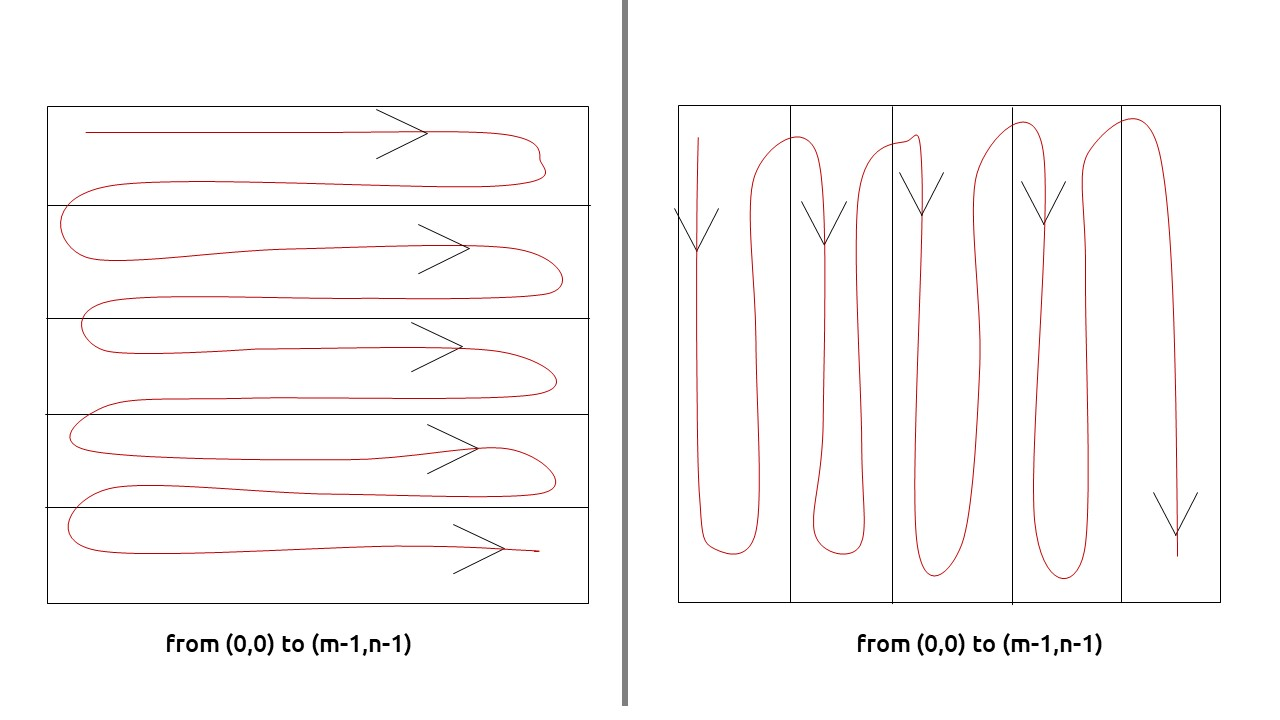• We can use two nested loops to have this traversal
• At every cell we calculate up and left as we had done in the recursive solution and then assign the cell’s value as (up+left)

Note: For the first row and first column (except for the top-left cell), then up and left values will be zero respectively.

Code:

## C++ Code

``````#include <bits/stdc++.h>

using namespace std;

int countWaysUtil(int m, int n, vector<vector<int> > &dp) {
for(int i=0; i<m ;i++){
for(int j=0; j<n; j++){

//base condition
if(i==0 && j==0){
dp[i][j]=1;
continue;
}

int up=0;
int left = 0;

if(i>0)
up = dp[i-1][j];
if(j>0)
left = dp[i][j-1];

dp[i][j] = up+left;
}
}

return dp[m-1][n-1];

}

int countWays(int m, int n){
vector<vector<int> > dp(m,vector<int>(n,-1));
return countWaysUtil(m,n,dp);

}

int main() {

int m=3;
int n=2;

cout<<countWays(m,n);
}
``````

Output:

3

Time Complexity: O(M*N)

Reason: There are two nested loops

Space Complexity: O(M*N)

Reason: We are using an external array of size ‘M*N’’.

## Java Code

``````import java.util.*;

class TUF{
static int countWaysUtil(int m, int n, int[][] dp) {

for(int i=0; i<m ;i++){
for(int j=0; j<n; j++){

//base condition
if(i==0 && j==0){
dp[i][j]=1;
continue;
}

int up=0;
int left = 0;

if(i>0)
up = dp[i-1][j];
if(j>0)
left = dp[i][j-1];

dp[i][j] = up+left;
}
}

return dp[m-1][n-1];

}

static int countWays(int m, int n){
int dp[][]=new int[m][n];
for (int[] row : dp)
Arrays.fill(row, -1);
return countWaysUtil(m,n,dp);

}

public static void main(String args[]) {

int m=3;
int n=2;

System.out.println(countWays(m,n));
}
}``````

Output:

3

Time Complexity: O(M*N)

Reason: There are two nested loops

Space Complexity: O(M*N)

Reason: We are using an external array of size ‘M*N’’.

Part 3: Space Optimization

If we closely look the relation,

dp[i][j] = dp[i-1][j] + dp[i][j-1])

We see that we only need the previous row and column, in order to calculate dp[i][j]. Therefore we can space optimize it.

Initially, we can take a dummy row ( say prev) and initialize it as 0.

Now the current row(say temp) only needs the previous row value and the current row’s value in order to calculate dp[i][j].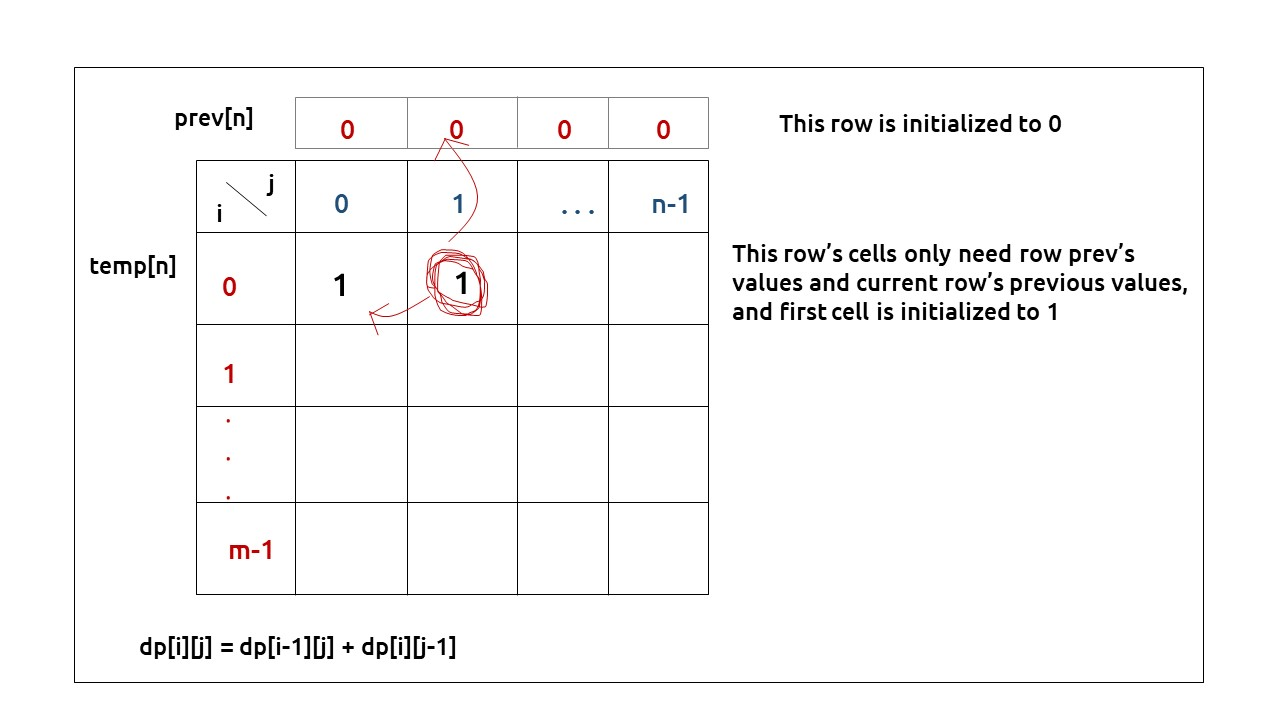At the next step, the temp array becomes the prev of the next step and using its values we can still calculate the next row’s values.At last prev[n-1] will give us the required answer.

Code:

## C++ Code

``````#include <bits/stdc++.h>

using namespace std;

int countWays(int m, int n){
vector<int> prev(n,0);
for(int i=0; i<m; i++){
vector<int> temp(n,0);
for(int j=0; j<n; j++){
if(i==0 && j==0){
temp[j]=1;
continue;
}

int up=0;
int left =0;

if(i>0)
up = prev[j];
if(j>0)
left = temp[j-1];

temp[j] = up + left;
}
prev = temp;
}

return prev[n-1];

}

int main() {

int m=3;
int n=2;

cout<<countWays(m,n);
}
``````

Output:

3

Time Complexity: O(M*N)

Reason: There are two nested loops

Space Complexity: O(N)

Reason: We are using an external array of size ‘N’ to store only one row.

## Java Code

``````import java.util.*;

class TUF{
static int countWays(int m, int n){
int prev[]=new int[n];
for(int i=0; i<m; i++){
int temp[]=new int[n];
for(int j=0; j<n; j++){
if(i==0 && j==0){
temp[j]=1;
continue;
}

int up=0;
int left =0;

if(i>0)
up = prev[j];
if(j>0)
left = temp[j-1];

temp[j] = up + left;
}
prev = temp;
}

return prev[n-1];

}

public static void main(String args[]) {

int m=3;
int n=2;

System.out.println(countWays(m,n));
}
}``````

Output:

3

Time Complexity: O(M*N)

Reason: There are two nested loops

Space Complexity: O(N)

Reason: We are using an external array of size ‘N’ to store only one row.